×#### Thank you for registering.

One of our academic counsellors will contact you within 1 working day.

Click to Chat

1800-1023-196

+91-120-4616500

CART 0

• 0

MY CART (5)

Use Coupon: CART20 and get 20% off on all online Study Material

ITEM
DETAILS
MRP
DISCOUNT
FINAL PRICE
Total Price: Rs.

There are no items in this cart.
Continue Shopping• Complete JEE Main/Advanced Course and Test Series
• OFFERED PRICE: Rs. 15,900
• View Details

```Chapter 21: Surface Area and Volume of a Sphere Exercise – 21.1

Question: 1

Find the surface area of a sphere of radius:

(i) 10.5 cm

(ii) 5.6 cm

(iii) 14 cm

Solution:

(i) Given Radius = 10.5 cm

Surface area = 4πr2

= 4 × 22/7 × (10.5)2

= 1386 cm2

(ii) Given radius = 5.6 cm

Surface area = 4πr2

= 4 × 22/7 × (5.6)2

= 394.24 cm2

(iii) Given radius = 14 cm

Surface area = 4πr2

= 4 × 22/7 × (14)2

= 2464 cm2

Question: 2

Find the surface area of a sphere of diameter:

(i) 14 cm

(ii) 21 cm

(iii) 3.5 cm

Solution:

(i) Given Diameter = 14 cm

= 14/2 = 7 cm

Surface area = 4πr2

= 4 × 22/7 × (7)2

= 616 cm2

(ii) Given Diameter = 21cm

= 21/2 = 10.5 cm

Surface area = 4πr2

= 4 × 22/7 × (10.5)2

= 1386 cm2

(iii) Given diameter = 3.5 cm

= 3.5/2 = 1.75 cm

Surface area = 4πr2

= 4 × 22/7 × (1.75)2

= 38.5 cm2

Question: 3

Find the total surface area of a hemisphere and a solid hemisphere each of radius 10cm (π = 3.14)

Solution:

The surface area of the hemisphere = 2πr2

= 2 × 3.14 × (10)2

= 628 cm2

The surface area of solid hemisphere = 2πr2

= 3 × 3.14 × (10)2

= 942 cm2

Question: 4

The surface area of a sphere is 5544 cm2, find its diameter.

Solution:

Surface area of a sphere is 5544 cm2

4πr2 = 5544

4 × 3.14 × (r)2 = 5544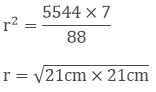r = 21cm

= 2(21) = 42 cm

Question: 5

A hemispherical bowl made of brass has inner diameter 10.5 cm. Find the cost of tin plating it on the inside at the rate of Rs.4 per 100 cm2.

Solution:

Inner diameter of hemispherical bowl = 10.5cm

Radius = 10.5/2 = 5.25 cm

Surface area of hemispherical bowl = 2πr2

= 2 × 3.14 × (5.25)2

= 173.25 cm2

Cost of tin plating 100cm2 area = Rs.4

Cost of tin plating 173.25 cm2Thus, the cost of tin plating the inner side of hemispherical bowl is Rs.6.93

Question: 6

The dome of a building is in the form of a hemisphere. Its radius is 63dm. Find the cost of painting it at the rate of Rs.2 per sq m.

Solution: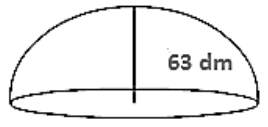Dome radius = 63 dm = 6.3 m

Inner surface area of dome = 2πr2

= 2 × 3.14 × (6.3)2

= 249.48 m2

Now, cost of 1m2 = Rs.2

Therefore cost of 249.48 m2 = Rs. (249.48 × 2) = Rs. 498.96

Question: 7

Assuming the earth to be a sphere of radius 6370 km, how many square kilometers is the area of the land if three-fourths of the earth’s surface is covered by water?

Solution:

3/4th of earth surface is covered by water

Therefore 1/4th of earth surface is covered by land

Therefore Surface area covered by land = 1/4 × 4πr2

= 1/4 × 4 × 22/7 × (6370)2

= 127527400 km2

Question: 8

A cylinder of same height and radius is placed on top of a hemisphere. Find the curved surface area of the shape if the length of the shape is 7cm.

Solution:

Given length of the shape = 7cmBut length = r + r

2r = 7cm

r = 3.5 cm

Also; h = r

Total surface area of shape = 2πrh + 2πr2

= 2πrr + 2πr2

= 2πr2 + 2πr2

= 4 × 22/7 × (3.5)2 = 154 cm2

Question: 9

A wooden toy is in the form of a cone surmounted on a hemisphere. The diameter of the base of the cone is 16 cm and its height is 15 cm. Find the cost of painting the toy at Rs.7 per 100 cm2

Solution:

Diameter of cone = 16 cmRadius of cone = 8 cm

Height of cone = 15 cm

Slant height of coneTherefore Total curved surface area of toy

= πrl + 2πr2

= 22/7 × 8 × 17 + 2 × 22/7 × 82

= 5808/7 cm2

Now, cost of 100cm2 = Rs.7

1cm2 = Rs. 7/100

Hence cost of 5808/7 cm2 = Rs. 5808/7 × 7/100

= Rs. 58.08

Question: 10

A storage tank consists of a circular cylinder with a hemisphere adjoined on either end. If the external diameter of the cylinder be 1.4 m and its length is 8 m, find the cost of painting it on the outside at the rate of Rs.10 per m2.

Solution: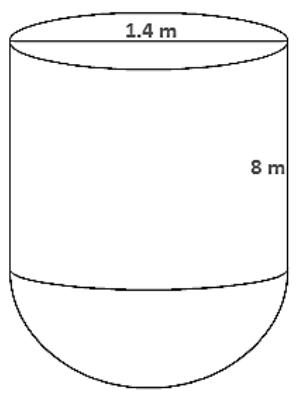Diameter of a cylinder = 1.4 m

Therefore radius of cylinder = 1.42 = 0.7 m

Height of cylinder = 8 m

Therefore surface area of tank = 2πrh + 2πr2

= 2 × 22/7 × 0.7 × 8 + 2 × 22/7 × (0.7)2

= 176/5 + 77/25 = 38.28 m2

Now cost of 1m2 = Rs.10

Therefore cost of 38.28 m2 = Rs. 382.80

Question: 11

The diameter of the moon is approximately one-fourth of the diameter of the earth. Find the ratio of their surface areas.

Solution:

Let the diameter of the earth be d

Then,

Diameter of moon will be d/4

Radius of moonSurface area of moon = 4π(d/8)2

Surface area of earth = 4π(d/2)2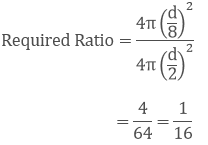Thus the required ratio of the surface areas is 1/16

Question: 12

A hemispherical dome of a building needs to be painted. If the circumference of the base of the dome is 17.6cm, find the cost of painting it, given the cost of painting is Rs.5 per 100 cm2

Solution:

Given that only the rounded surface of the dome to be painted, we would need to find the curved surface area of the hemisphere to know the extent of painting that needs to be done.

Now, circumference of the dome = 17.6 cm

Therefore 2πr = 17.6

2 × 22/7 × r = 17.6 m

So, the radius of the dome = 2πr2

= 2 × 22/7 × 2.8 × 2.8

= 49.28m2

Cost of painting 100 cm2 is Rs.5

So, the cost of painting 1m2 = Rs.500

Therefore the cost of painting the whole dome = Rs. 500 × 49.28

= Rs. 24640

Question: 13

The front compound wall of a house is decorated by wooden spheres of diameter 21 cm, placed on small supports as shown in the figure. Eight such spheres are used for this purpose and are to be painted silver. Each support is a cylinder of radius 1.5 cm and height 7cm and is to be painted black. Find the cost of paint required if silver paint costs 25 paise per cm2.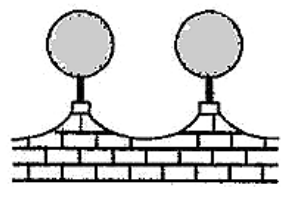Solution:

Wooden sphere radius = 21/2 = 10.5 cm

Surface area of a wooden sphere = 4πr2 = 4 × 22/7 × (10.5)2 = 1386 cm2

Radius r of cylindrical support = 1.5 cm

Height h of cylindrical support = 7cm

Curved surface area of cylindrical support = 2πrh = 2 × 22/7 × 1.5 × 7 = 66 cm2

Area of circular end of cylindrical support = πr2 = 22/7 × (1.5)2 = 7.07cm2

Area to be painted silver = 8(1386 − 7.07) cm2

= 8(1378.93) cm2

= 11031.44 cm2

Cost occurred in painting silver colour = (11034.44 × 0.25) = Rs.2757.86

Area to be painted black = (8 × 66) cm2 = 528 cm2

Cost occurred in painting black colour = (528 × 5.05) = Rs.26.40

Therefore total cost in painting = Rs.2757.86 + Rs.26.40

= Rs.2784.26
```### Course Features

• 728 Video Lectures
• Revision Notes
• Previous Year Papers
• Mind Map
• Study Planner
• NCERT Solutions
• Discussion Forum
• Test paper with Video Solution